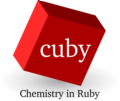back to Modules

# cuby_neb

This module implements the Nudged Elastic Band (NEB) method for identification of minimum energy path between two minima.

Since the starting points on the path are prepared by interpolation between reactant and product, it is useful to define the structure in internal coordinates (z-matrix) with the same topology where the interpolation produces meaningful structures.

The calculation optimizes simultaneously the geometries of multiple points along the minimum energy path, the result of the calculation are these geometries and their respective energies.

Current implementation is just a proof of concept, possible improvement include:

• Better optimizer
• Parallel calculation of images

The module requires two geometries to be specified:

``cuby_neb input.yaml reactant.zmat product.zmat``

### Example

Simple calculation of energy barrier for rotation of methyl group in propane can be found in the examples directory as #04.

### Keywords

NEB setup

Geometry optimization: